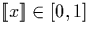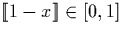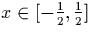Next: Relationship between cons' and Up: Useful Properties Previous: Useful Properties

### Range Analysis

It is often possible to analyse the range of a finite portion of given stream, and use this information to determine output digits for an algorithm computing some operation applied to this stream. For example, suppose we are computing (1-x), and we know that x is of the form (1::x'), we can say that, and hence. We can represent all reals in this range using a stream starting with the digit one, and so we can output this digit without examining further digits of x. It was thus possible to generate one output digit by examining only one input digit. The example given is a specific case, but this general principle may be applied in numerous ways.

In some cases we can extend this technique as follows. Suppose we know by range analysis that, but that it need not necessarily start with the digit zero. If we wish to compute 2x, a legal operation given the range of x, the simplest way is to perform a left shift by removing a zero from the head of the stream representing x. Obviously, however, this method will not work if the first digit of x is not a zero. Fortunately we can define a function which will re-represent the stream starting with a specified digit, in this case zero, which we can then remove. This function for signed binary streams is described in section 4.1.4, and is referred to in this work as the p' function.Next: Relationship between `cons' and Up: Useful Properties Previous: Useful Properties
Martin Escardo
5/11/2000